×

×

TULANE / Chemistry / CHEM 1070 / What is Balmer's equation?

# What is Balmer's equation? Description

##### Description: Packed with hints and shortcuts from each section, including the most important facts to remember, and practice problems with step-by-step solutions! Enjoy
18 Pages 8 Views 10 Unlocks
Reviews

Bernita Feeney (Rating: )

If Nina isn't already a tutor, they should be. Haven't had any of this stuff explained to me as clearly as this was. I appreciate the help!

Chemistry Fall Final 2015: Study Guide!

## What is Balmer's equation?Balancing Oxidation-Reduction Reduction:

In acidic solution:

Step 1: Split the reaction into 2 half reactions

Step 2: Balance the elements that aren't oxygen or hydrogen

Step 3: Balance oxygens by adding water

Step 4: Balance hydrogens by adding protons (H+)

Step 5: Balance charges by adding electrons

Step 6: Scale the equations so that electrons will cancel out when you combine half reactions Step 7: Combine half reactions and cancel out common terms

In basic solution:

*Follow steps 1-7 as in acidic solution

Step 8: Add OH- to cancel out H+

Step 9: Combine OH- and H+ to form water

Example 1) Balance MnO4- + I- ===> Mn2+ + I2 in acidic solution

Step 1: MnO4- ===> Mn2+

I- =====> I2

Step 2: MnO4- ===> Mn2+

2I- ====> I2

Step 3: MnO4- ===> Mn2+ + 4H2O

## What is diamagnetic?2I- ====> I2

Step 4: 8H+ +MnO4- ===> Mn2+ + 4H2O

2I- ====> I2

Step 5: 5e- + 8H2O + MnO4- ===> Mn2+ + 4H2O

2I- ====> I2 + 2e

Step 6: 2*(5e- + 8H2O + MnO4- ===> Mn2+ + 4H2O)

5*(2I- ====> I2 + 2e-)

Step 7: 16H+ + 2MnO4- + 10I- ===> 2Mn2+ + 8H2O + 5I2

Example 2) Balance ClO2 ===> ClO3- + Cl

Step 1: ClO2 ===> ClO3-

ClO2 ===> Cl

Step 2: ClO2 ===> ClO3-

ClO2 ===> Cl

Step 3: H2O + ClO2 ===> ClO3-

ClO2 ===> Cl- + 2H2O

Step 4: H2O + ClO2 ===> ClO3- + 2H+

4H+ + ClO2 ===> Cl- + 2H2O

Step 5: H2O + ClO2 ===> ClO3- + 2H+ 1e

5e- + 4H+ + ClO2 ===> Cl- + 2H2O

Step 6: 5*(H2O + ClO2===> ClO3- + 2H+ + 1e-)

5e- + 4H+ + ClO2 ===> Cl- + 2H2O

Step 7: 6ClO2 + 3H2O ===> 5ClO3- + 6H+ + Cl We also discuss several other topics like subjectable

Step 8: 6OH- + 6ClO2 + 3H2O ===> 6OH- + 5ClO3- + 6H+ + Cl

## The number of molecular orbitals formed is equal to the number of atomic orbitals combined.Step 9: 6OH- + 6ClO2 ===> 5ClO3- + 3H2O + Cl-

Limiting Reagents:

Limiting reagents are the reactants in a reaction that stop the reaction first. This happens  because the amount of this reactant will run out before the other reactant(s). To find the limiting  reagent, you must pay close attention to the moles of each reactant that are required in a chemical  equation. For example, in the equation 2 AgI + Na2S → Ag2S + 2 NaI, if you have 3 moles of AgI  and 4 moles of Na2S, the AgI would be the limiting reagent, because to have both reactants react  completely, you would need 2 moles of AgI to every 1 mole of Na2S, and in this case that would  mean you'd need 8 moles of AgI.

Example 1) A 0.696 mol sample of Cu is added to 136 mL of 6.0 M HNO3. Assuming the following  reaction is the only one that occurs, will the Cu react completely?

3 Cu(s) + 8HNO3(aq) ----> 3 Cu(NO3)2(aq) + 4H2O(l) + 2NO(g)

What we really need to find out: Is Cu or HNO3 the limiting reagent?

What we know: We need 3 moles of Cu to every 8 moles of HNO3

We have 0.696 moles of Cu and 136 mL of 6.0 M HNO3 If you want to learn more check out asu class override

Step 1: Convert 136 mL of 6.0 M HNO3 into moles

136 mL*(1L/1000mL)*(6 mole/1L)= 0.816 moles HNO3

Step 2: See if ratio of moles Cu to moles HNO3 is equal to 3:8

3(x)= 0.696 moles Cu—-> x= 0.232

8(x)= # moles HNO3 we need to complete reaction

8(0.232)= 1.856 moles HNO3, which is greater than 0.816

Answer: No, we do not have enough HNO3 for Cu to react completely

Example 2: A side reaction in the manufacture of rayon from wood pulp is

3 CS2 + 6 NaOH ----> 2 Na2CS3 + Na2CO3 + 3H2O

How many grams of Na2CS3 are produced in the reaction of 92.5 mL of liquid CS2 (d=1.26 g/mL)  and 2.78 mol NaOH?

What we really need to find out: Which is the limiting reagent? This will tell us how  many moles of Na2CS3 we can produce with these amounts of reactants, and then we can convert  that to grams.

What we know: We need 3 moles of CS2 to every 6 moles of NaOH

We have 92.5 mL of liquid CS2 which has a density of 1.26 g/mL

and 2.78 moles of NaOH

The complete reaction will produce 2 moles of Na2CS3 to every 3

moles CS2 and every 6 moles NaOH

Step 1: Convert 9.25 mL of CS2 into moles

92.5 mL*(1.26 g/mL)*(1 mole/76 g)= 1.533 mole CS

Step 2: See if ratio of moles CS2 to moles NaOH is equal to 3:6

3(x)= 1.533 moles CS2 ----> x= 0.511

6(0.511)= 3.066 moles NaOH, which is greater than 2.78 moles NaOH that  we have, which means that NaOH is the limiting reagent

6(z)= 2.78 moles NaOH ----> z= 0.463

2(z)= # moles Na2CS3 that can be produced

2(0.463)= 0.926 moles Na2CS3

0.926 moles Na2CS3*(154 g/1 mole)= 142.6

Answer: We will produce 142.6 grams of Na2CS3

Calorimetry:  If you want to learn more check out How long should protections last and is it promoting or limiting progress?

Important points to know:

1 calorie= 4.184 Joule If you want to learn more check out how do you say discuss in spanish

Specific heat of water (heat capacity of water) = (4.18J/ (gram of water*degree Celsius)) quantity of heat= q = mass of substance*specific heat*temperature change

= heat capacity*temperature change

qsystem= -qsurroundings

qreaction= -qcalorimeter

qcalorimeter = heat capacity of calorimeter*temperature change

Change in internal energy= ΔU= q (heat) + w (work)= Ufinal – Uinitial

If reaction occurs at constant volume: ΔU= qrxn + w= qrxn + 0= qv

Enthalpy = H= ΔU + ΔPV

If a process is carried out under constant temperature and pressure and with work limited to  pressure-volume work, then ΔH= ΔU + PΔV and heat flow is ΔH= qp Don't forget about the age old question of a wimba wep

Hess's Law: If a process occurs in stages or steps, the enthalpy change for the overall process is the  sum of the enthalpy changes for the individual steps

Translation: If you carry out a reaction in more than one steps, the sum of the  enthalpy changes of those steps will equal the enthalpy change of the reaction if you did it in one  step.

Enthalpies of formation: The standard enthalpy of formation of a pure element in its reference form  is 0.

Standard enthalpy change: ΔH^o

ΔH^o= (sum of ΔHf^o(products)*vp) – (sum of ΔHf^o(reactants)*vr)

v= stoichiometric coefficients (number that comes before molecule in rxn equation)

PV= nRT

R= .08206 L atm/ mol K

Practice Problems:

1) Calculate the quantity of heat, in kilojoules, required to raise the temperature 9.25 L water from  22.0 to 29.4 degrees Celsius

What we need to know: How many grams of water do we have? What is the temperature  change?

What we know: We have 9.25 L of water

Water is going from 22.0 to 29.4 degrees Celsius

Specific heat of water is 4.18 Joules/(g*degree C)

Step 1: Convert water to grams

9.25 L*(1000 mL/1 L)*(1 g/1 mL)= 9250 g

Step 2: Find temperature change

29.4-22.0= 7.4 degrees Celsius

Step 3: Use specific heat of water to find quantity of heat in Joules

9250 g*7.4 degrees C*(4.18 Joules/(g*degrees C))= 286121 Joules Don't forget about the age old question of if a 35 percent increase in price of golf balls led to an 42 percent decrease in quantity demanded, then the demand for golf balls is

Step 4: Convert to kilojoules

286121 Joules*(1 kJ/1000 J)= 286.121 kJ

Calorimetry Practice Continued:

2) A 74.8 g sample of copper at 143.2 degrees Celsius is added to an insulated vessel containing 165  mL of glycerol, C3H8O3(l) (d= 1.26 g/mL), 24.8 degrees C. The final temperature is 31.1 degrees  Celsius. The specific heat of copper is 0.385 Joules/(g*degree C). What is the specific heat of  glycerol in Joules/(mole*degree C)?

What we know: We have 74.8 g sample of copper and 165 mL of glycerol

The specific heat of copper is 0.385 Joules/(g*degree C)

The temperature goes from 143.2 to 31.1 degrees Celsius for copper, and

from 24.8 to 31.1 degrees Celsius for glycerol

qcopper= qglycerol

density of glycerol is 1.26 grams per mL

There are 420 grams per mole of glycerol

What we need to know: Amount of glycerol in grams

Temperature change for each substance

Step 1: Convert glycerol to grams

165 mL*(1.26 g/mL)= 207.9 g glycerol

Step 2: Find temperature change for each substance

Copper: 31.1-143.2= -112.1 degrees Celsius---> ΔT= 112.1

Glycerol: 31.1- 24.8= 6.3 degrees Celsius ---> ΔT= 6.3

Step 3: Solve for heat capacity of glycerol using equations for q

qcopper= qglycerol

mass of copper*specific heat of copper*temperature change of copper= mass  of glycerol*specific heat of glycerol*temperature change of glycerol

74.8 g*0.385 Joules/(g*degree C)*112.1 degrees C= 207.9 g

glycerol*specific heat of glycerol*6.3 degrees

Specific heat of glycerol= 2.645 Joules/(g*degree C)

Step 4: Convert from Joules/(g*degree C) to Joules/(mole*degree C)

2.645 Joules/(g*degree C)*(420 g/1mole)= 226.78 Joules/(mole*degree C)

Answer: The specific heat of glycerol is 226.78 Joules/(mole*degree C)

3) The combustion of methane gas, the principal constituent of natural gas, is represented by the  equation

CH4(g) + 2 O2(g) ------> CO2(g) + 2 H2O(l) ΔHo= -890.3 kJ

What mas of methane, in kilograms, must be burned to liberate 2.80*107 kJ of heat? What we know: Burning 1 mole of methane will produce 890.3 kJ of heat

There are 16 grams in every 1 mole of methane

What we need to know: The ratio of 2.80*107 kJ to 890.3 kJ

Step 1: Find out how many times 890.3 goes into 2.80*107 kJ

2.80*107 kJ/ 890.3= 31450.07

Step 2: Convert moles CH4 to grams

31450.07 moles methane*(16 grams/1 mole)= 503201.17 g methane

Step 3: Convert grams to kilograms

503201.17 g methane*(1 kg/1000 g)= 503.20117 kg

Answer: Must burn 503 kg of methane to liberate 2.80*107 kJ of heat

Light-energy-wave/particle problems

Important things to remember:

1 meter= 106 micrometers, 109 nm (nanometer), 1012 pm (picometer)

C= speed of light= 3*108 meters/sec

C= υ*λ υ= frequency--> units are Hz=1/sec λ= wavelength--> units are metersPlanck's constant: h=6.626*10-34 Joules*sec

E= Energy per photon= h*υ

p= momentum= (h*υ)/C= h/λ -----> λ= (h)/(m*u) (m=mass, u= velocity)

mass of electron= 9.109*10-31 kg

Molecular mass=M= (m/V) (RT/P)

Balmer's equation: υ= 3.2881*1015 s^-1((1/22)- (1/n2))

Bohr atom description equations:

rn= n2ao

ao= 53 picometers

En= Energy of an electron at a level= (-RH)/(n2) RH= 2.179*10-18

ΔE= RH((1/ni2)-(1/nf2)) where ni= initial level and nf= final level

*only works in hydrogen-like species with only 1 electron; for other species: En= (-Z2RH)/(n2)

Uncertainty principle: Δx= uncertainty in position, Δp= uncertainty in momentum  ΔxΔp≥ h/(4π)

Δp= m*Δu----->Δu= uncertainty of velocity

Wave mechanics:

λ= 2L/n L= length of “string”, n+1= total number of nodes

Ek= Energy of wave= (n2h2)/8mL2

Quantum numbers:

n= principal quantum number= 1,2,3,4,....

(integers starting at 1 and increasing)

tells you what the orbital is: orbital number=n

l = orbital angular momentum quantum number= 0,1,2,3,...n-l

(can't be larger than n-l)

tells you what subshell type is: l=0=s, l=1=p, l=2=d, l=3=f

ml = magnetic quantum number= -l, (-l+1),...,(l-1),+l

(starting at -l and going to positive l up by 1)

corresponds to number of orbitals in each subshell (s:n=1,l=0, ml=0-->only 1 orbital)

ms: electron spin quantum number ( + ½, - ½)

each orbital can have up to 1 electron that is + ½ (↑) and one that is – ½ (↓)

can't have [↑↑] or [↓↓]

Energy level

Sublevels

# of orbitals

# of electrons

1

s

1

2

2

s,p

1,3= 4

8

3

s,p,d

1,3,5=9

18

4

s,p,d,f

1,3,5,7=16

32

Aufbau process: *fill in the s subshell first

*can't have electrons of the same spin in an orbital

Ground state: if all orbitals have at least one electron, they do [↑ ][↑ ][↑ ]

Excited state: if all orbitals could have at least one electron, one has moved [↑↓][↑ ][ ]

Total number of nodes= n-1

# angular nodes= l

Practice Problems:

1) Use the Balmer equation to determine

a) the frequency, in s-1, of the radiation corresponding to n=5

b) the wavelength, in nanometers, of the line in the Balmer series corresponding to n=7 c) the value of n corresponding to the Balmer series line at 380 nm

a) The frequency, in s-1, of the radiation corresponding to n=5

Step 1: plug n=5 into Balmer's equation

υ= 3.2881*1015 s-1 ((1/22) – (1/52))= 6.90501*1014 s-1

b) The wavelength, in nanometers, of the line in the Balmer series corresponding to n=7 What we need to know: The frequency of the radiation corresponding to n=7 What we know: C= λυ---> λ= C/υ

C= 3*108 meters/sec

Step 1: Plug in the Balmer equation to λ= C/υ

λ= C/(3.2881*1015 s-1 ((1/22) – (1/n2)))

λ= (3*108)/(3.2881*1015 s-1 ((1/22) – (1/n2)))

Step 2: Plug n=7 into the new equation

λ= (3*108)/(3.2881*1015 s-1 ((1/22) – (1/72)))= 3.97*10-7

Step 3: Convert the wavelength from meters to nanometers

3.97*10-7*(109 nm/1m)= 397.4 nanometers

c) The value of n corresponding to the Balmer series line at 380 nm

What we need to know: What frequency corresponds to the wavelength of 380nm? What we know: C= λυ---> υ= C/λ

C= 3*108 meters/sec

Step 1: Convert frequency from nanometers to meters

380 nm*(1m/ 109 nm)= 3.8*10-7 m

Step 2: Use the equation υ= C/λ to find frequency

υ= (3*108)/(3.8*10-7)= 7.89*1014

Step 3: Use Balmer's equation to solve for n

υ= 7.89*1014= 3.2881*1015((1/22) – (1/n2))

n=10

2) A certain radiation has a wavelength of 574 nm. What is the energy, in Joules, of a) one photon

b) a mole of photons of this radiation

a) A certain radiation has a wavelength of 574 nm. What is the energy, in Joules, of one photon? What we need to know: The frequency corresponding to this wavelength

What we know: E= hυ

υ= C/λ

E= (hC)/λ

λ= 574 nm

Step 1: Convert wavelength from nanometers to meters

λ= 574 nm*(1 m/109 nm)= 5.74*10-7 meters

Step 2: Plug in wavelength to E= (hC)/λ equation

E= (6.626*10-34*3*108)/ 5.74*10-7= 3.463*10-19 Joules/photon

b) A certain radiation has a wavelength of 574 nm. What is the energy, in Joules, of a mole of  photons of this radiation?

What we know: 1 mole= 6*1023

Step1: Convert Joules/photon to Joules/mole

3.463*10-19 Joules/photon*(6*1023 photons/1 mole)= 207,783.9721 Joules/mole

3) Use the description of the Bohr atom in the text to determine

a) the radius, in nanometers, of the sixth Bohr orbit for hydrogen

b) the energy, in Joules, of the electron when it is in this orbit

a) Use the description of the Bohr atom in the text to determine the radius, in nanometers, of the  sixth Bohr orbit for hydrogen.

What we know: rn= n2ao

n=6

ao= 53 picometers

1 m= 1012 pm= 109 nm

Step 1: Convert picometers to nanometers

53 pm*(1m/1012 pm)*(109 nm/1 m)= 0.053 nm

Step 2: Plug in ao, now in nanometers, into rn equation

r6= 62*0.053= 1.098 nm

Answer: Radius of the sixth Bohr orbit for hydrogen equals 1.098 nm

b) Use the description of the Bohr atom in the text to determine the energy, in Joules, of the electron  when it is in this orbit.

What we know: En= (-RH)/(n2)

RH= 2.179*10-18 Joules

n=6

Step 1: Plug in the given n and RH to the energy equation

E6= (-2.179*10-18)/(62)= -6.05*10-20 Joules

Answer: The energy of an electron in the 6th Bohr orbit is -6.05*10-20 Joules

5) What must be the velocity, in meters per second, of a beam of electrons if they are to display a de  Broglie wavelength of 1micrometer?

What we know: 1 meter= 106 micrometers

λ= (h)/(m*u)

velocity of beam of electrons=velocity of one electron in that beam

mass of electron= 9.109*10-31 kg

h= 6.626*10-34

Step 1: Convert wavelength from micrometers to meters

λ= 1 micrometer*(1 m/106 micrometers)= 10-6 meters

Step 2: Plug in wavelength in meters, h, and mass of electron to the equation λ= (h)/(m*u)  and solve for u

10-6= (6.626*10-34)/(9.109*10-31*u)---> u= 727 m/s

Answer: The velocity of a beam of electrons that display a de Broglie

wavelength of 1 micrometer is 727 m/s

6) A proton is accelerated to one-tenth the velocity of light, and this velocity can be measured with a  precision of 1%. What is the uncertainty in the position of this proton?

What we know: ΔxΔp≥ h/(4π)-->Δp=uncertainty of momentum, Δx= uncertainty of position  Δp= m*Δu----->Δu= uncertainty of velocity

C= speed of light= 3*108 m/s

uncertainty of something= value*(% precision/100)

mass of proton= 1.67*1027 kg

h= 6.626*10-34

Step 1: Find velocity of proton

u= (1/10*C)= (1/10)*(3*108)= 30,000,000 m/s

Step 2: Find the uncertainty of the velocity

Δu= 30,000,000*(1/100)= 30,000

Step 3: Find uncertainty of momentum

Δp= m*Δu= (1.67*10-27)*(30,000)= 5.01*10-22

Step 4: Plug in uncertainty of momentum and h into ΔxΔp≥ h/(4π) and solve for Δx Δx*(5.01*10-22)= (6.636*10-34)/(4π)---> Δx= 1.05*10-13 m

Answer: The uncertainty in the position of the proton is 1.05*10-13 meters

7) What type of orbital (ie, 3s, 4p,...) is designated by these quantum numbers? a) n=5, l= 1, ml= 0

b) n=4, l= 2, ml= -2

c) n= 2, l= 0, ml= 0

a) What type of orbital (ie, 3s, 4p,...) is designated by n=5, l= 1, ml= 0?

What we know: n tells you what the orbital is: orbital number=n

tells you what subshell type is: l=0=s, l=1=p, l=2=d, l=3=f

Answer: n=5, l= 1, ml= 0 is the orbital 5p

b) What type of orbital (ie, 3s, 4p,...) is designated by n=4, l= 2, ml= -2?

What we know: n tells you what the orbital is: orbital number=n

tells you what subshell type is: l=0=s, l=1=p, l=2=d, l=3=f

Answer: n=4, l= 2, ml= -2 is the orbital 4d

c) What type of orbital (ie, 3s, 4p,...) is designated by n= 2, l= 0, ml= 0?

What we know: n tells you what the orbital is: orbital number=n

tells you what subshell type is: l=0=s, l=1=p, l=2=d, l=3=f

Answer: n= 2, l= 0, ml= 0 is the orbital 2s

8) Concerning the electrons in the shells, subshells, and orbitals of an atom, how many can have a) n=4, l=2, ml= 1, ms= + ½

b) n=4, l= 2, ml= 1

c) n=4

d) n=4, l=2, ms= + ½

a) Concerning the electrons in the shells, subshells, and orbitals of an atom, how many can have  n=4, l=2, ml= 1, ms= + ½?

What we know: n tells you what the orbital is: orbital number=n

tells you what subshell type is: l=0=s, l=1=p, l=2=d, l=3=f

ml = magnetic quantum number= -l, (-l+1),...,(l-1),+l

corresponds to number of orbitals in each subshell (s:n=1,l=0,

ml=0-->only 1 orbital)

each orbital can have up to 1 electron that is + ½ (↑) and one that is – ½ (↓) Step 1: Determine orbital number

orbital #=n=4

subshell type==> l=2==> subshell d==> 4d

Step 3: Find orbital number in subshell

l= 2==> ml could be: -2,-1,0,1,2---> ml= 1= 4th magnetic quantum #---> 4d^4 Step 4: Check electron spin

ms= + ½ -----> only 1

Answer: Only 1 can have n=4, l=2, ml= 1, ms= + ½

b) Concerning the electrons in the shells, subshells, and orbitals of an atom, how many can have  n=4, l=2, ml= 1, ms= + ½?

What we know: n tells you what the orbital is: orbital number=n

tells you what subshell type is: l=0=s, l=1=p, l=2=d, l=3=f

ml = magnetic quantum number= -l, (-l+1),...,(l-1),+l

corresponds to number of orbitals in each subshell (s:n=1,l=0,

ml=0-->only 1 orbital)

each orbital can have up to 1 electron that is + ½ (↑) and one that is – ½ (↓) Step 1: Determine orbital number

orbital #=n=4

subshell type==> l=2==> subshell d==> 4d

Step 3: Find orbital number in subshell

l= 2==> ml could be: -2,-1,0,1,2---> ml= 1= 4th magnetic quantum #---> 4d^4

Can have 2 in each orbital slot

Answer: 2 can have n=4, l=2, ml= 1

c) Concerning the electrons in the shells, subshells, and orbitals of an atom, how many can have  n=4, l=2?

What we know: n tells you what the orbital is: orbital number=n

tells you what subshell type is: l=0=s, l=1=p, l=2=d, l=3=f

ml = magnetic quantum number= -l, (-l+1),...,(l-1),+l

corresponds to number of orbitals in each subshell (s:n=1,l=0,

ml=0-->only 1 orbital)

each orbital can have up to 1 electron that is + ½ (↑) and one that is – ½ (↓) Step 1: Determine orbital number

orbital #=n=4

subshell type==> l=2==> subshell d==> 4d

d subshell has 5 orbitals and can therefore have 10 electrons

Answer: 10 can have n=4, l=2

d) Concerning the electrons in the shells, subshells, and orbitals of an atom, how many can have  n=4?

What we know: n tells you what the orbital is: orbital number=n

tells you what subshell type is: l=0=s, l=1=p, l=2=d, l=3=f

ml = magnetic quantum number= -l, (-l+1),...,(l-1),+l

corresponds to number of orbitals in each subshell (s:n=1,l=0,

ml=0-->only 1 orbital)

each orbital can have up to 1 electron that is + ½ (↑) and one that is – ½ (↓) Step 1: Determine orbital number

orbital #=n=4

4 can have subshells s,p,d,f--> respectively, 1,3,5,7 orbitals= total of 32 electrons Answer: 32 can have n=4

e) Concerning the electrons in the shells, subshells, and orbitals of an atom, how many can have  n=4, l=2, ms= + ½?

What we know: n tells you what the orbital is: orbital number=n

tells you what subshell type is: l=0=s, l=1=p, l=2=d, l=3=f

ml = magnetic quantum number= -l, (-l+1),...,(l-1),+l

corresponds to number of orbitals in each subshell (s:n=1,l=0,

ml=0-->only 1 orbital)

each orbital can have up to 1 electron that is + ½ (↑) and one that is – ½ (↓) Step 1: Determine orbital number

orbital #=n=4

subshell type==> l=2==> subshell d==> 4d--> 5 orbitals

Step 3: Check electron spin

ms= + ½ -----> only one electron per orbital

Answer: 5 can have n=4, l=2, ms= + ½

9) Identify the orbital that has

a) 1 radial node, 1 angular node

b) no radial nodes, 2 angular nodes

c) 2 radial nodes, 3 angular nodes

a) Identify the orbital that has 1 radial node, 1 angular node What we know: Total number of nodes= n-1

# angular nodes= l

Step 1: Find n

n-1= total number of nodes= 1+1= 2 ----> n= 3 Step 2: Find l

l= number of angular nodes= 1

Step 3: Find subshell type

l=1 ----> subshell type p

b) Identify the orbital that has no radial nodes, 2 angular node What we know: Total number of nodes= n-1

# angular nodes= l

Step 1: Find n

n-1= total number of nodes= 0+2= 2 ----> n= 3 Step 2: Find l

l= number of angular nodes= 2

Step 3: Find subshell type

l=2 ----> subshell type d

c) Identify the orbital that has no radial nodes, 2 angular node What we know: Total number of nodes= n-1

# angular nodes= l

Step 1: Find n

n-1= total number of nodes= 2+3= 5 ----> n= 6 Step 2: Find l

l= number of angular nodes= 3

Step 3: Find subshell type

l=3 ----> subshell type f

10) What is the expected ground-state electron configuration for each of the following elements? a) mercury

b) calcium

c) polonium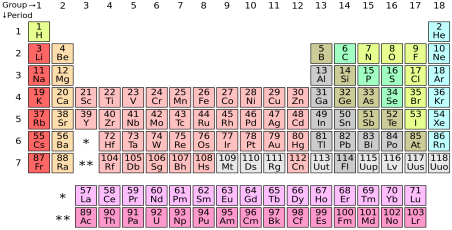a) What is the expected ground-state electron configuration for mercury? Step 1: Identify the noble gas that precedes the element

Xenon---> [Xe]

Step 2: Identify first subshell of electrons that should be added

always s subshell

6th period---> 6s

goes past s subshell--> 6s2

[Xe] 6s2

Step 3: Identify the next subshell of electrons that should be added

6th period--> 4f subshell, which has 14 electrons

goes past f subshell--> 4f14

[Xe] 6s2 4f14

Step 4: Identify the next subshell of electrons that should be added

6th period--> 5d subshell, which has 10 electrons

Hg is in the last spot of d orbital--> 5d10

b) What is the expected ground-state electron configuration for calcium?

Step 1: Identify the noble gas that precedes the element

Argon---> [Ar]

Step 2: Identify first subshell of electrons that should be added

always s subshell

4th period---> 4s

s subshell has 2 electrons

Ca at end of 4s subshell--> 4s2

c) What is the expected ground-state electron configuration for polonium? Step 1: Identify the noble gas that precedes the element

Xenon---> [Xe]

Step 2: Identify first subshell of electrons that should be added

always s subshell

6th period---> 6s

goes past s subshell--> 6s2

[Xe] 6s2

Step 3: Identify the next subshell of electrons that should be added

6th period--> 4f subshell, which has 14 electrons

goes past f subshell--> 4f14

[Xe] 6s2 4f14

Step 4: Identify the next subshell of electrons that should be added

6th period--> 5d subshell, which has 10 electrons

goes past 5d subshell--> [Xe] 6s2 4f14 5d10

Step 5: Identify the next subshell of electrons that should be added

6th period--> 6p subshell, which has 6 electrons

Po is in 4th spot in 6p subshell--> 6p4

Answer: [Xe] 6s2 4f14 5d10 6p4

11) Between which two orbits of the Bohr hydrogen atom must an electron fall to produce light of  wavelength 1876 nm?

What we know: ΔE= RH((1/ni2)-(1/nf2))

RH= 2.179*10-18

E= hC/λ

h= 6.626*10-34

C= 3*108

n's must be positive integer values greater than 0

Step 1: Convert wavelength from nanometers to meters

1876 nm*(1 m/108 nm)= 1.876*10-6 meters

Step 2: Plug in C, h, and wavelength to E= hC/λ

E= (6.626*10-34*3*108)/(1.876*10-6)= 1.06*10-19 Joules

Step 3: Plug in E and RH into ΔE= RH((1/ni2)-(1/nf2))

1.06*10-19 Joules= 2.179*10-18*((1/ni2)-(1/nf2))

(1/ni2)-(1/nf2)= 0.0486276

Step 4: Guess and check values for ni and nf

(1/22)-(1/32)==> too large of a value

(1/32)-(1/42)= 0.0486276

Lewis Structures, resonance, VSEPR

Important things to remember:

Atomic radius increases from right to left and top to bottom on periodic table Cations (positive ions) are smaller than the atoms from which they are formed Anions are larger than the atoms from which they are formed

Isoelectronic ions have identical electron configurations

Ionization energy and Electron Affinity increase from left to right and from bottom to top on  periodic table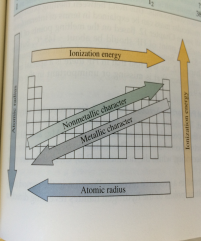Diamagnetic: All electrons are paired

Paramagnetic: Unpaired electrons exist

Practice Problems:

1) For each of the following pairs, indicate the atom that has the larger size: a) Te or Br

b) K or Ca

c) Ca or Cs

d) N or O

e) O or P

f) Al or Au

a) Te b) K c) Cs d) N e) P f) Au

2) Among the following ions, several pairs are isoelectronic. Identify these pairs: Fe2+, Sc3+, Ca2+, F-, Co2+, Co3+, Sr2+, Cu^+, Zn2+, Al3+

Fe2+ and Co3+ (both Cr), Sc3+ and Ca2+ (both Ar), F- and Al3+ (both Ne), Cu+ and Zn2+ (both Ni)

3) Unpaired electrons are found in only one of the following species. Indicate which one: F-, Ca2+, Fe2+, S2-

F-: 2s2 2p5 + 1e-= 8 e- ====> [↑↓] [↑↓][↑↓][↑↓]

Ca2+: 4s2 – 2e-= 0 e- ====> [ ]

Fe2+: 4s2 3d6 – 2e-= 6 e- ====> [↑↓] [↑ ][↑ ][↑ ][↑ ][ ]

S2-: 3s2 3p4 + 2e- = 8e- ====> [↑↓] [↑↓][↑↓][↑↓]

Number of  electron

groups

Electron

group

geometry

Number of  lone pairs

VSEPR

Notation

(A=central)

Molecular  geometry

Ideal Bond  Angles

(degrees)

Example

2

Linear

0

AX2

Linear

180

BeCl2

3

Trigonal

planar

0

AX3

Trigonal

planar

120

BF3

3

Trigonal

planar

1

AX2E

Bent

120

SO2

4

Tetrahedral

0

AX4

Tetrahedral

109.5

CH4

4

Tetrahedral

1

AX3E

Trigonal

pyramidal

109.5

NH3

4

Tetrahedral

2

AX2E2

Bent

109.5

OH2

5

Trigonal

bipyramidal

0

AX5

Trigonal

bipyramidal

90, 120

PCl5

5

Trigonal

bipyramidal

1

AX4E

Seesaw

90, 120

SF4

5

Trigonal

bipyramidal

2

AX3E2

T-shaped

90

ClF3

5

Trigonal

bipyramidal

3

AX2E3

Linear

180

XeF2

6

Octahedral

0

AX6

Octahedral

90

SF6

6

Octahedral

1

AX5E

Square

pyramidal

90

BrF5

6

Octahedral

2

AX4E2

Square

planar

90

XeF4

4) Each of the following molecules contains at least one multiple covalent bond. Give a plausible  Lewis Structure for

a) OCS

b) CH3CHO

c) F2CO

d) Cl2SO

e) C2H2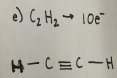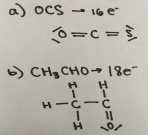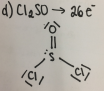5) The following polyatomic anions involve covalent bonds between O atoms and the central  nonmetal atom. Propose an acceptable Lewis Structure for each:

a) SO32-

b) NO2-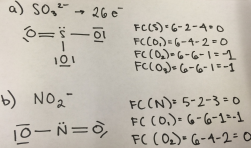Orbital hybridization:

Important things to remember:

The number of hybrid orbitals equal the total number of atomic orbitals that are combined' sp: linear (2 electron groups)

sp2: trigonal planar (3 electron groups)

sp3: tetrahedral (4 electron groups)

sp3d: trigonal bipyramidal (5 electron groups)

sp3d2: octahedral (6 electron groups)

sigma vs pi bonds:

first bond between two atoms is always sigma

preceding bonds are pi

if two atoms are triple bonded, there is 1 sigma bond and 2 pi bonds

*pay attention to positive and negative signs on orbitals

Molecular Orbitals:

The number of molecular orbitals formed is equal to the number of atomic orbitals  combined

When two atomic orbitals are combined, one of the molecular orbitals is a bonding orbital at  a lower energy, and one is an anti-bonding orbital at a higher energy

In ground state configurations, electrons enter the lowest energy molecular orbit available The maximum number of electrons in a molecular orbital is two

In ground-state configurations, electrons enter molecular orbits of identical energies singly  before they pair up

s orbital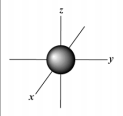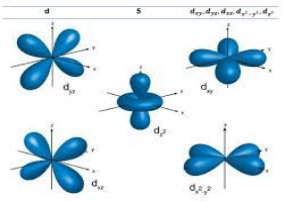from top to right: Px, Pz, Py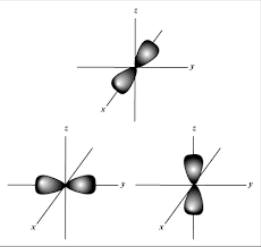Page ExpiredIt looks like your free minutes have expired! Lucky for you we have all the content you need, just sign up here
References: